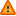FAQ/reglineR - CBU statistics WikiYou should trust both wikis because the password could be read by the particular administrators.

Clear message
location: FAQ / reglineR

# Putting user defined regression lines on scatterplots in R

One example where you may wish to insert two or more regression lines on a scatterplot is when you have groups whose line of best fit you wish to plot assuming they have the same slope (ie as assumed in an ANCOVA).

This is also covered in the regression graduate statistics talk and demos. The code below extends the R syntax described in the graduate talk by specifying the minimum and maximum values on the axes using xlim and ylim options in the plot command and in placement of the legend outside of the plot. R is particularly good at legends for describing regression lines for different groups because it produces a legend which succinctly describes both marker and line information to denote the different groups which is important for displaying graphical results in monochrome.

The code below takes a SPSS data file (R PLOTS FORMAT2.sav) containing four columns denoting the Raven score (predictors Raven1 and Raven2) and WCST values (responses WCST1 and WCST2) for each of two groups (patients (1) and controls (2)) and plots the two regression lines (one for each group) which, as assumed in ANCOVA, have the same slope but different intercepts. The values of these coefficients can be obtained as regression coefficients from a linear regression using group and Raven to predict WCST and then entered into the plot using the 'abline' function.

This first set of code puts the legend in the plotting area like this. The jpeg command sends the outputted graph into a portable jpeg file you can send via e-mail.

```library(foreign)
jpeg("U:\\My Documents\\My Documents2\\JOHN D ANCOVA FOR WIKI\\WCST.jpeg")
x <- read.spss("C:\\Documents and Settings\\peterw\\Desktop\\My Documents\\My Documents2\\JOHN D ANCOVA FOR WIKI\\JD PLOTS 25-1-12\\R PLOTS FORMAT2.sav")
attach(x)
plot(Raven1,WCST1,xlim=c(10,40),ylim=c(1,6),pch=1,,xlab="RCPM(Total Score)",ylab="WCST (Number of categories achieved)")
points(Raven2,WCST2,pch=4)
abline(a=-0.455,b=0.183,lty=2)
abline(a=-0.181,b=0.183,lty=1)
legend(x=28,y=2,c("Controls","Patients"), pch=c(4,1),lty=c(1,2))```

You can also split a vector, Raven, into two, Raven1 and Raven2, using the split function to split the vector into Ravens score for each of the groups whose regression lines you wish to plot. The code for this is below.

```rgp <- split(Raven, Group)
attach(rgp)```

The Ravens scores will then be put into separate columns (for Patients and Controls) each beginning with rgp and succeeded by the label name (if any) which was used in the SPSS spreadsheet to describe the group codings e.g. rgp\$'PD PATIENTS' and rgp\$CONTROLS give the Raven data for the patients and controls respectively in this example since 'PD PATIENTS' and CONTROLS were used to label the respective group codings in the SPSS spreadsheet. These data columns then be used in the above syntax to represent predictor scores in each of the two groups for plotting their regression lines assuming equal slopes. The below R syntax plots regression lines with the legend in the plotting area after splitting each of the Ravens and WCST scores into two columns, one for each group.

```rgp <- split(Raven, Group)
wgp <- split(WCST, Group)
attach(rgp)
attach(wgp)
jpeg("U:\\My Documents\\My Documents2\\JOHN D ANCOVA FOR WIKI\\WCST.jpeg")
plot(rgp\$CONTROLS,wgp\$CONTROLS,xlim=c(10,40),ylim=c(1,6),pch=1,xlab="RCPM(Total Score)",ylab="WCST (Number of categories achieved)")
points(rgp\$'PD PATIENTS',wgp\$'PD PATIENTS',pch=4)
abline(a=-0.455,b=0.183,lty=2)
abline(a=-0.181,b=0.183,lty=1)
legend(x=28,y=2,c("Controls","Patients"), pch=c(4,1),lty=c(1,2))```

The code below will put the legend outside (and to the right of) the plot like this. This graph is slightly smaller than the one with the legend in the plotting area to accommodate the extra space needed for the legend.

```library(foreign)
jpeg("U:\\My Documents\\My Documents2\\JOHN D ANCOVA FOR WIKI\\WCST.jpeg")
x <- read.spss("C:\\Documents and Settings\\peterw\\Desktop\\My Documents\\My Documents2\\JOHN D ANCOVA FOR WIKI\\JD PLOTS 25-1-12\\R PLOTS FORMAT2.sav")
attach(x)
par(xpd=TRUE, mar=par()\$mar+c(0,0,0,4))
plot(Raven1,WCST1,xlim=c(10,40),ylim=c(1,6),pch=1,xlab="RCPM(Total Score)",ylab="WCST (Number of categories achieved)")
points(Raven2,WCST2,pch=4)
legend(x=41,y=5,c("Controls","Patients"), pch=c(4,1),lty=c(1,2),bty='n')
par(xpd=FALSE)
abline(a=-0.455,b=0.183,lty=2)
abline(a=-0.181,b=0.183,lty=1)```

A slight variant of the above examples produces the plot axes minus the points and adds each group on separately afterwards instead of adding the points in the first group on first. The below example also shows you can add more than two groups onto the ANCOVA plot (in this case three).

```jpeg("U:\\My Documents\\My Documents2\\JOHN D ANCOVA FOR WIKI\\WCST.jpeg")
plot(PCA5_PC1,WCST,type="n",xlim=c(-3,3),ylim=c(1,6),xlab="gGTB",ylab="WCST (Number of categories achieved)")
#plot(PCA5_PC1,WCST,xlab="gGTB",ylab="WCST (Number of categories achieved)")
points(PCA5_PC11,WCST1,pch=1)
points(PCA5_PC12,WCST2,pch=4)
points(PCA5_PC13,WCST3,pch=5)
abline(a=4.607,b=0.737,lty=2)
abline(a=3.141,b=0.737,lty=1)
abline(a=4.113,b=0.737,lty=3)
legend(x=1,y=2,c("Controls","LFftd","HFftd"), pch=c(1,4,5),lty=c(2,1,3))```

Not the col=n (where n is a positive integer) subcommand can be used with points,legend and abline to create coloured points and lines e.g. col=1 is black, col=2 is red, col=3 is green and col=4 is blue.

The below uses raw data input creating a group variable in R to identify the two groups during plotting and saves to a .eps file which hs a higher resolution that .jpeg. R can save graphs in other formats - see here.

```library(foreign)
x <- read.spss("U:\\My Documents\\My Documents2\\JOHN D ANCOVA FOR WIKI\\JD PLOTS 25-1-12\\Todrawgraphs.sav")
attach(x)
group1 <- x\$Group=="PD PATIENTS"
group2 <- x\$Group=="CONTROLS"
dev.new()
plot(Raven,WCST,type="n",xlim=c(10,40),ylim=c(1,6))
points(Raven[group1=="TRUE"],WCST[group1=="TRUE"],pch=1,col=1)
points(Raven[group2=="TRUE"],WCST[group2=="TRUE"],pch=4,col=2)
abline(a=-0.455,b=0.183,lty=2,col=1)
abline(a=-0.181,b=0.183,lty=1,col=2)
legend(x=28,y=2,c("Controls","Patients"), pch=c(4,1),lty=c(1,2),col=c(2,1))
dev.print(device=postscript, "U://My Documents//graph1.eps", onefile=FALSE, horizontal=FALSE)
dev.off()```